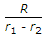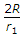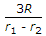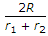# Civil Engineering - Applied Mechanics

### Exercise :: Applied Mechanics - Section 2

26.

A spring scale in a stationary lift shows a reading of 60 kg for a man standing on it. If the lift starts descending at an acceleration of g/5, the scale reading would be

 A. 48 kg B. 60 kg C. 72 kg D. none of these.

Explanation:

No answer description available for this question. Let us discuss.

27.

The resolved part of the resultant of two forces inclined at an angle θ in a given direction is

 A. algebraic sum of the resolved parts of the forces in the direction B. arithmetical sum of the resolved parts of the forces in the direction C. difference of the forces multiplied by cosine θ° D. sum of the forces multiplied by the sine θ E. sum of the forces multiplied by the tangent θ°.

Explanation:

No answer description available for this question. Let us discuss.

28.

The velocity ratio of the differential wheel and axle is

 A.B.C.D.Explanation:

No answer description available for this question. Let us discuss.

29.

The following statement is one of the laws of Dynamic friction

 A. The force of friction always acts in a direction opposite to that in which a body is moving B. The magnitude of the kinetic friction bears a constant ratio to the normal reaction between two surfaces. The ratio being slightly less than that in the case of limiting friction C. For moderate speeds the force of friction remains constant but decreases slightly with the increase of speed D. all the above.

Explanation:

No answer description available for this question. Let us discuss.

30.

A 50 kg boy climbs up a 8 m rope in gymnasiam in 10 sec. The average power developed by the boy is approximately

 A. 400 watts B. 500 watts C. 4000 watts D. none of these.